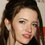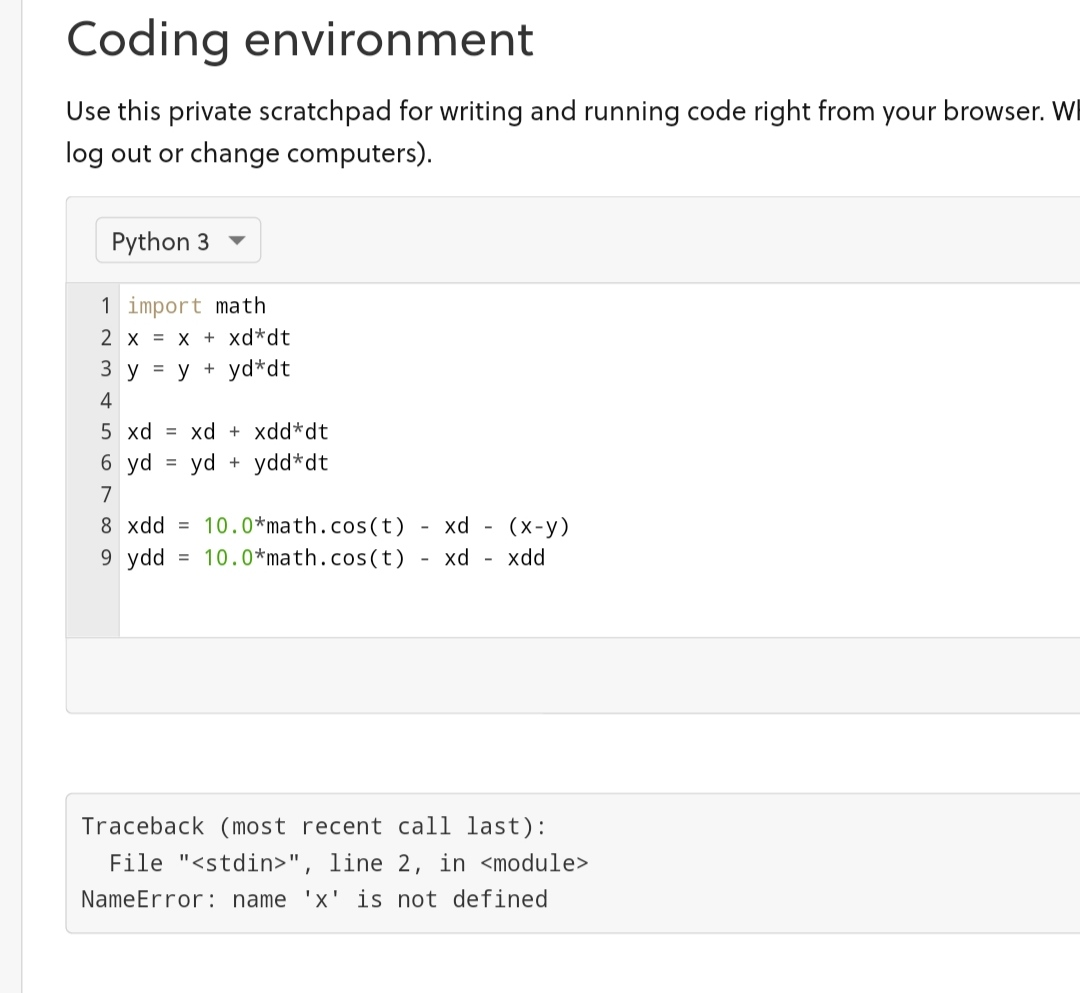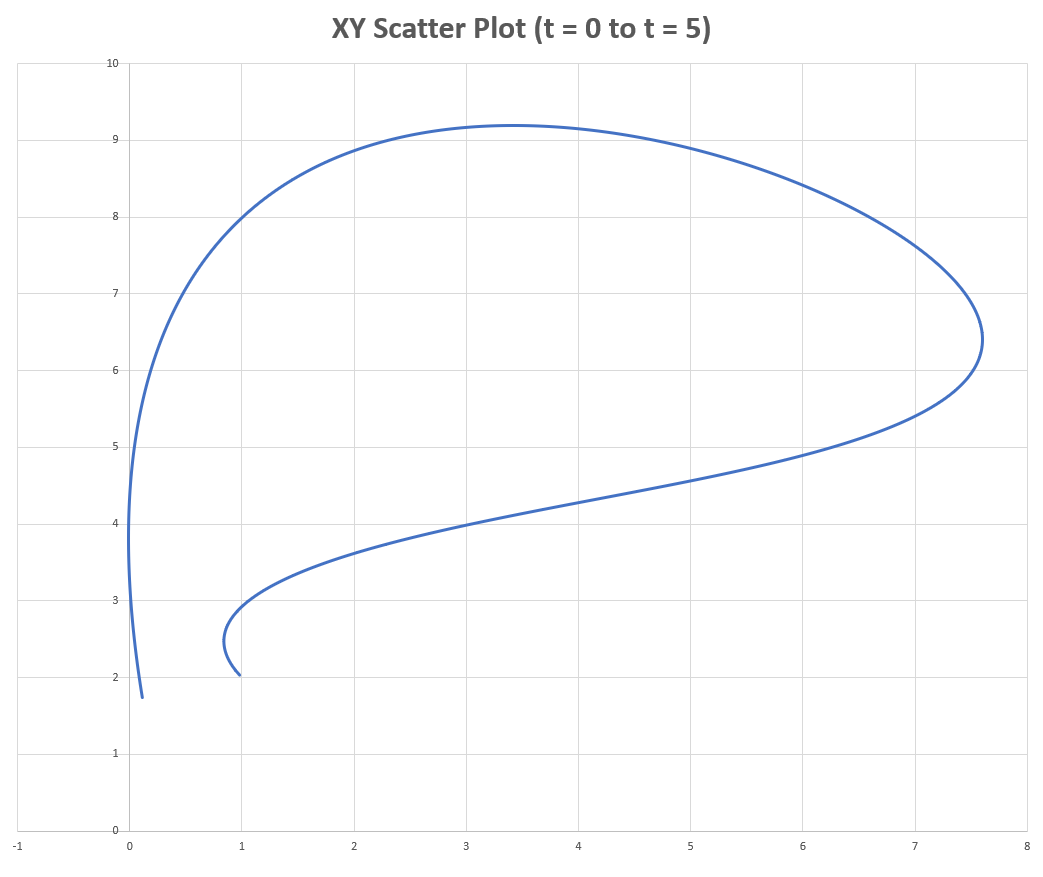# Differential Equations

I was solving this particular pair of differential equation.
Both $$x$$ and $$y$$ are function of $$t$$(time) $10 \cos t-\dot{x}-\ddot{x}-\ddot{y}=0$
$10 \cos t-\dot{x}-\ddot{x}-(x-y) =0$
Where $\dot{p}$ and $\ddot{p}$ are single and double derivaties of $p$ with respect to $t$ (time) , respectively.

Is there any general form of solution, or some technique through Laplace transformation.
Any help will be appreciated.Note by Talulah Riley
10 months, 2 weeks ago

This discussion board is a place to discuss our Daily Challenges and the math and science related to those challenges. Explanations are more than just a solution — they should explain the steps and thinking strategies that you used to obtain the solution. Comments should further the discussion of math and science.

When posting on Brilliant:

• Use the emojis to react to an explanation, whether you're congratulating a job well done , or just really confused .
• Ask specific questions about the challenge or the steps in somebody's explanation. Well-posed questions can add a lot to the discussion, but posting "I don't understand!" doesn't help anyone.
• Try to contribute something new to the discussion, whether it is an extension, generalization or other idea related to the challenge.

MarkdownAppears as
*italics* or _italics_ italics
**bold** or __bold__ bold
- bulleted- list
• bulleted
• list
1. numbered2. list
1. numbered
2. list
Note: you must add a full line of space before and after lists for them to show up correctly
paragraph 1paragraph 2

paragraph 1

paragraph 2

[example link](https://brilliant.org)example link
> This is a quote
This is a quote
    # I indented these lines
# 4 spaces, and now they show
# up as a code block.

print "hello world"
# I indented these lines
# 4 spaces, and now they show
# up as a code block.

print "hello world"
MathAppears as
Remember to wrap math in $$ ... $$ or $ ... $ to ensure proper formatting.
2 \times 3 $2 \times 3$
2^{34} $2^{34}$
a_{i-1} $a_{i-1}$
\frac{2}{3} $\frac{2}{3}$
\sqrt{2} $\sqrt{2}$
\sum_{i=1}^3 $\sum_{i=1}^3$
\sin \theta $\sin \theta$
\boxed{123} $\boxed{123}$

Sort by:

- 10 months, 2 weeks ago

For something this complex, your best bet is probably numerical integration. Here is how it would look in Python (explicit Euler)

 1 2 3 4 5 6 7 8 x = x + xd*dt y = y + yd*dt xd = xd + xdd*dt yd = yd + ydd*dt xdd = 10.0*math.cos(t) - xd - (x-y) ydd = 10.0*math.cos(t) - xd - xdd 

- 10 months, 2 weeks ago

@Steven Chase Sir the code is not working- 10 months, 2 weeks ago

Yeah, it requires more code to actually run. I'll post the complete code soon

- 10 months, 2 weeks ago

@Steven Chase by the way I am very curious to know that how I will get $x$ and $y$ as a function of time because, python always gives results in numerical answer .
So I am very excited see that how python will do it?

- 10 months, 2 weeks ago

@Lil Doug Some differential equations can't be written as a function of time, like this one. This one is a nonlinear second order ODE; it is therefore extremely hard (or even impossible) to write a function of time of $x$.

Take the pendulum with drag:

$\ddot{\theta} = -g \sin(\theta) + Cl\dot{\theta}$

There are (as of now) no analytical solutions for the differential equation, as to the function $\theta$. The one you've posted above is much harder than the pendulum equation already.

- 10 months, 2 weeks ago

@Steven Chase sir can you post a python based solution of my latest problem.
Hope I am not disturbing you.

- 10 months, 1 week ago

Hello. It is up now

- 10 months, 1 week ago

Here is the full code, with some initialized values and plotting.

  1 2 3 4 5 6 7 8 9 10 11 12 13 14 15 16 17 18 19 20 21 22 23 24 25 26 27 28 29 30 31 32 33 34 35 36 import math dt = 10.0**(-5.0) ################################################## t = 0.0 count = 0 x = 1.0 y = 2.0 xd = -2.0 yd = 3.0 xdd = 10.0*math.cos(t) - xd - (x-y) ydd = 10.0*math.cos(t) - xd - xdd ################################################## while t <= 5.0: x = x + xd*dt y = y + yd*dt xd = xd + xdd*dt yd = yd + ydd*dt xdd = 10.0*math.cos(t) - xd - (x-y) ydd = 10.0*math.cos(t) - xd - xdd t = t + dt count = count + 1 if count % 1000 == 0: print t,x,y- 10 months, 2 weeks ago

I want to know; some people do Explicit Euler in an inverted order; acceleration, velocity, then position, but you've done it in the opposite way. Is there a major difference in the two orders?

- 10 months, 2 weeks ago

I doubt it makes too much difference. I basically taught myself how to do these things. So it wouldn't surprise me if my style was a bit unorthodox.

- 10 months, 2 weeks ago

@Steven Chase Do you use time-domain simulation as part of your day-to-day engineering?

- 10 months, 2 weeks ago

I do indeed. For simple things, I use little hand-crafted state-space simulations. For larger and more complex applications, we have more sophisticated (and much more expensive) time-domain simulation tools.

- 10 months, 2 weeks ago

@Steven Chase have a look on last 5 hour notifications

- 10 months, 2 weeks ago

@Steven Chase How do you choose this values of $x, \dot{x}, y, \dot{y}$ are all these with random, or these value are basically a set, which are folloing the pair of differential equations.

- 10 months, 2 weeks ago

You can initialize the position and velocity any way you want. And then the initial accelerations are determined by the differential equations.

- 10 months, 2 weeks ago

@Steven Chase But how
$x=5, \dot{x}=6, y=7, \dot{y}=8$
So these are my assumed values, now how to find acceleration?

- 10 months, 2 weeks ago

Re-arrange your second equation to solve for the x acceleration. Then plug that into your first equation and re-arrange to solve for the y acceleration.

- 10 months, 2 weeks ago

@Lil Doug If you have any questions about Explicit Euler, ask me, if Steven Chase's busy.

- 10 months, 2 weeks ago

I think he is lazy instead of busy.

- 10 months, 2 weeks ago

@Lil Doug Lol; just ask me your question. If it's about differential equations or numerical solving, I'm sure I can answer it. I taught myself that stuff a while ago, like him.

- 10 months, 2 weeks ago

@Krishna Karthik Thanks
By the way, I have posted a mechanics problem, don't forget to solve and post a solution

- 10 months, 2 weeks ago

@Steven Chase who said that the value of x is 1 ??
I am again very much curious to know your method

- 10 months, 2 weeks ago

I just chose some values to initialize the simulation with

- 10 months, 2 weeks ago